# Descriptive Statistics in ExcelArticle byExcelMojo Team## What Is Descriptive Statistics In Excel?

Descriptive statistics in Excel summarizes and organizes the values of a given dataset. The values can be a collection of opinions or observations. The function simplifies lengthy worksheets and enables users to understand the data better.

The quantitative analysis of the data set is calculated by describing the relationship between one variable with another. In this sense, Excel has the following formulas to perform descriptive statistical calculations:

1. Frequency Measures
• MIN, MAX, COUNT, Frequency, and Percent functions show how often a value occurs in the dataset.
1. Central Tendency Measures
• Mode, Mean, and Median functions to find the distribution by various points.
1. Dispersion or Variation Measures
• Variance, Range, and Standard Deviation mainly show how data dispersion affects the data set’s mean. For instance, the range will show us the highs and lows of the data set.

Likewise, we can perform a whole set of descriptive statistics in Excel using the Analysis ToolPak.

###### Key Takeaways
• Descriptive statistics in excel allows users to get many statistical calculations in just a click, saving a lot of time.
• Analysis ToolPak is a hidden feature in Excel. We need to enable it to start using descriptive statistics in excel.
• Users should know the function and how the formula works to interpret the descriptive statistical result or have a statistical background.
• We can perform each descriptive statistical calculation using individual formulas like MIN, MAX, STDEV.S, AVERAGE, etc.

### How To Enable Descriptive Statistics?

Descriptive Statistics in excel is a built-in feature provided by Microsoft to perform a lot of descriptive data analysis. You have already enabled descriptive statistics in Excel if you can see ‘Data Analysis’ under the ‘Data’ tab.

However, if you do not see this under the ‘Data’ tab, follow the below steps to enable it.

1. Step 1: Click on the File tab.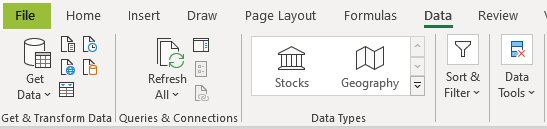2. Select Options.3. Once you select ‘Options,’ the ‘Excel Options’ window pops up. Choose ‘Add-ins’ in the window as highlighted below.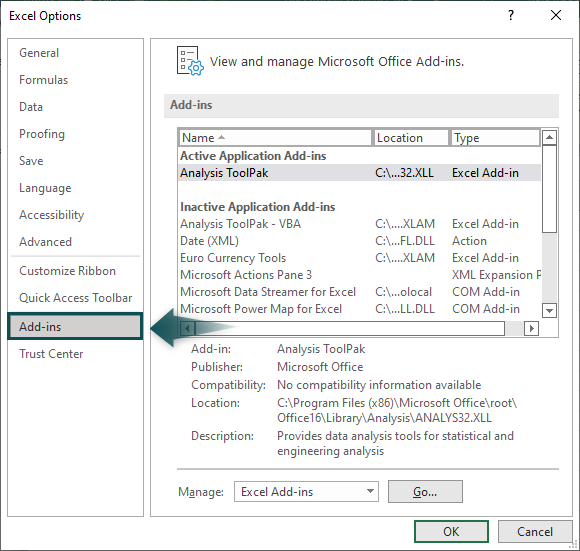4. Click on Excel Add-ins from the ‘Manage’ drop-down.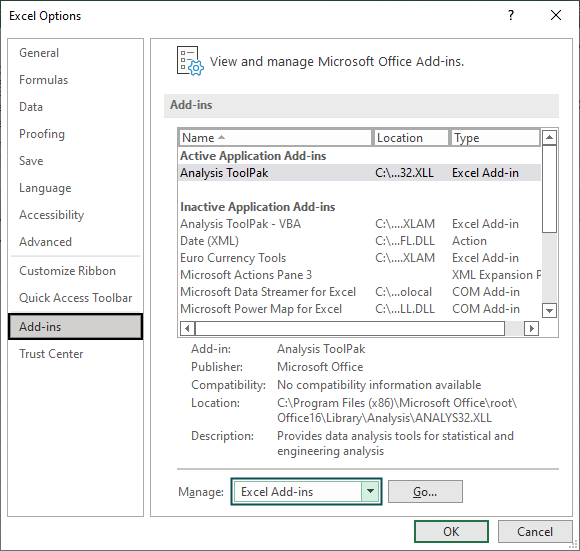5. Click on the Go tab. You can see a list of available add-ins options. Choose ‘Analysis ToolPak.’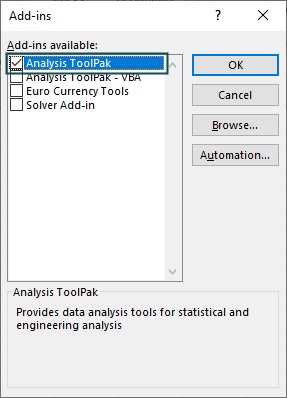Click OK to enable the Data Analysis option under the Data tab.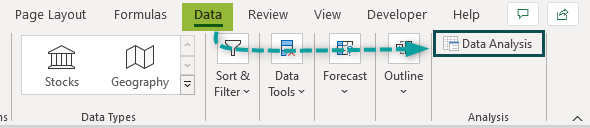### How To Use Descriptive Statistics?

Following is the table with a list of scores obtained by students in an examination.

We will run descriptive statistics in Excel with the following steps:

Step 1: Click on the ‘Data’ tab.

Step 2: Select the ‘Data Analysis’ option under the Data tab.

Step 3: The ‘Data Analysis’ window with a list of ‘Analysis Tools’ options appears. Choose ‘Descriptive Statistics’ and click OK.

Step 4: The Descriptive Statistics window pops up.

Step 5: For Input Range, choose the Score column, i.e., the cell range B2:B11.

Since we have selected the input range without headers, we can ignore the Labels in First Row check box.

Step 6: For Output Range, choose the cell where we want to place our descriptive statistics result.

Step 7: Select any one of the statistics options as required. We can also choose all four options in Excel.

Step 8: Click OK to get the results using descriptive statistics in excel.

As shown in the above image, we have obtained all kinds of descriptive statistics for the given data (students’ scores). The highest score (largest value) is 97, and the lowest (smallest value) is 42.

Thus, we can calculate descriptive statistics in Excel using Analysis ToolPak to summarize the dataset.

### Examples

Let us look at some advanced descriptive statistics in Excel examples for clear understanding:

#### Example #1: Descriptive Statistics of Human Resources, Including the Headers

Consider the following human resource data of a textile company. We have all the company employees’ salary, age, and gender information in an Excel spreadsheet.

Let us use the above data set to find descriptive statistics in excel in the following steps:

Step 1: Click the ‘Data’ tab.

Step 2: Select the ‘Data Analysis’ option under the ‘Data‘ tab.

Step 3: The ‘Data Analysis’ window with a list of ‘Analysis Tools’ options appears. Choose ‘Descriptive Statistics’ and click OK.

Step 4: The ‘Descriptive Statistics’ window pops up.

Step 5: For Input Range, choose the Salary column, i.e., the cell range B1:B9.

Step 6: We have selected the data range, including the headers. So, we need to check the Labels in the first-row box as highlighted below.

Step 7: For Output Range, choose cell F1.

Step 8: Check all the statistics options.

Once we select all the options, we can change the default values.

• Confidence Level for Mean: By default, this is 95%. We can change the confidence level for the mean according to our requirements.
• Kth Largest: By default, this will be 1, i.e., the 1st largest value from the given data set. We can change the default value. For instance, changing the value to 5 will return the 5th highest value from the data set.
• Kth Smallest: By default, this will be 1, i.e., the 1st smallest value from the given data set. We can change the default value to any other number. For instance, changing the value from 1 to 3 will display the 3rd lowest value from the data set.

Now click OK to find descriptive statistics in excel.

Interpretation of the Result: As shown in the above table, the Descriptive Analysis feature has simplified the dataset by providing the required calculations. Let us learn the formula and the steps involved in calculating the following functions for better understanding:

• Mean in cell G3: Mean is the average value of the data. In this example, the average salary of all the employees (from A2:A9) is displayed in cell G3 as \$6,646. It is calculated using the formula:

Total Salary / Total Number of Employees

• Median in cell G5: Median is the middle number of the given data set, arranged in ascending or descending order. In this example, the median value arranged in ascending order is \$7,171.
• Standard Deviation in cell G7: This is very important in statistics. The standard deviation in excel shows how the data is dispersed or spread. The higher the deviation, the higher the variability of the data points. The standard deviation is \$2,692, so the employees’ salary ranges from \$4,000 to \$9,200.

The formula to calculate Standard Deviation is:

Mean +/- of Standard Deviation.

• Minimum in cell G12: The MIN function returns the lowest value from the data. In our example, the lowest salary among all the employees is \$3,298.
• Maximum in cell G13: The MAX function returns the highest data value. In this example, the highest salary among all the employees is returned as \$9,869.

Range in cell G11: The Range function determines the difference between the highest and the lowest values. The difference between the highest and lowest salaries is: \$9,869 – \$3,298 = \$6,571.

• Count: The COUNT function counts the total number of values in the data. The total number of records included in this example is eight employees.

#### Example #2: Descriptive Statistics of New Product Feedback Survey

The following table shows the data of customers based on a survey of the newly launched products.

Let us calculate descriptive statistics in excel for both Age (in column D) and Survey Score (in column E).

Step 1: Select the ‘Data’ tab and click on the ‘Data Analysis’ option.

Step 2: For ‘Input Range,’ choose both Age and Survey Score, i.e., the cell range D1:E17.

Step 3: Since we have selected the headers of two columns, D and E, in the Input Range, we need to check the Labels in first row box.

Step 4: Select a cell reference (H1) in the Output Range to display the results.

Click OK to obtain the descriptive statistics for the dataset.

As shown in the image above, Excel has provided the descriptive statistics for both Age and Survey Score.

The average age of the customers who have participated in the survey is 55.68, and the average survey score is 61.62.

The standard deviation of the age is 13.5, indicating that the age distribution is plus or minus either side of the mean value of 55.68, i.e., 42 to 68. So the age difference is not that significant in this example.

The standard deviation of the survey score is 19.18, so this indicates that the distribution of data is plus or minus either side of the mean value of 61.65, i.e., 43 to 80. There is a widespread distribution of survey scores.

Likewise, we can perform various statistical calculations in just a click by using descriptive statistics in Excel.

### Important Things To Note

• Descriptive statistics is a built-in Excel feature. The feature is default hidden, but one can enable it under Add-ins.
• It works only for the numerical data set.
• Descriptive statistics result based on the confidence level for mean by default is 95%.
• When we select the header of the data set, we must check the Labels in first row box to get accurate results.

How to Perform Descriptive Statistics in Excel?

First, we need to enable Analysis ToolPak to run descriptive statistics in Excel. For example, let us consider the below table with a set of scores.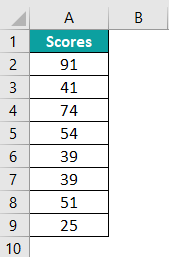Go to the Data tab and click on the ‘Data Analysis’ option.

The ‘Data Analysis’ window pops up with Analysis Tools options. Choose Descriptive Statistics from the available options.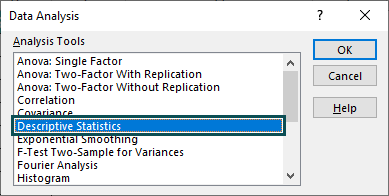Now for Input Range, choose cell range A2:A9.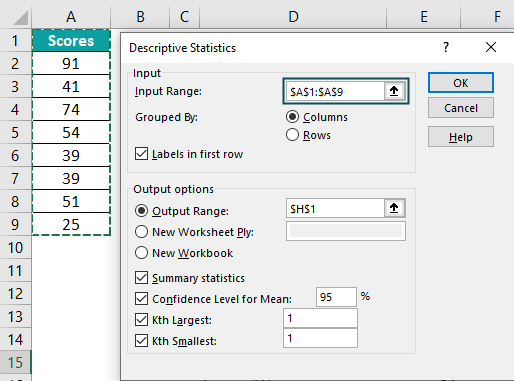For the Output Range, choose cell D1.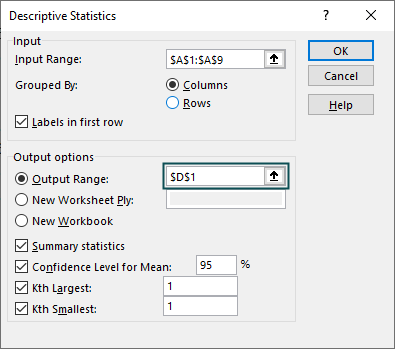Click OK to obtain the results using the Descriptive Analysis feature.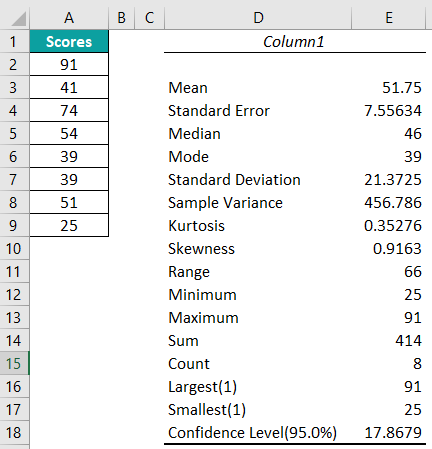Where is Descriptive Statistics in Excel?

Descriptive statistics in Excel is a hidden feature, and one has to enable it by using the below steps.

Step 1: Choose File >>> Options >>> Add-insChoose Analysis ToolPak in the ‘Add-ins‘ window.Click OK to see the Data Analysis option under the Data tab.How to Interpret Descriptive Statistics Results in Excel?

Descriptive statistics interpretation is based on statistical knowledge. Following are a few interpretation points to remember.

– We can look at the minimum and maximum values from the data set.
– The mean is the average of the data set, and the standard deviation should be added and deducted from the mean value to find the distribution of the data set. The higher the standard deviation, the greater the chances of data being distributed widely.
– The range is the difference between the maximum and minimum values.
– The count is the total number of records considered for statistical analysis.